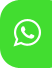## Product Detail## Ignou Solved Assignment for BMTC-131 | IGNOU Solved Assignment B.Sc Mathematics

University  IGNOU
Service Type Solved Assignment
Course B.Sc(Mathematics)
Semester
Short Name or Subject Code B.Sc (Mathematics) BMTC-131
Product B.Sc(Mathematics) of Solved Assignment (IGNOU)
Pattern
Price
Click to view price

PART – A

1. Which of the following statements are true, and which are false? Give reasons for your answers in the form of a short proof or a counterexample.

i) There are at least two ways of describing the set ,8,7{ . ...}

ii) Any function with domain R×R is a binary operation.

iii) The graph of every function from ]1,0[ to R is infinite.

iv) The function :f R → R , defined by )x(f = x x , is an odd function.

v) The domain of the function ,g f o where )x(f = x and )x(g = 2 − ,x is ]2, ]− ∞ .

2. a) Obtain the 6th roots of )7 (− , and represent them in an Argand diagram.

b) Using the ε −δ definition, show that lim 3( x )5 1 x 2 − = → .

3. a) Find the nature of the roots of the polynomial 2x x 5x 1 5 3 + + + .

b) Let      − > − ≤ < − < = x( )3 if, x 3 3 x if, 0 x 3 x if, x 0

i) Check whether f is discontinuous. If yes, find where?

ii) Give a rough sketch of the graph of f.

c) Express the function + → + :g R R , defined by , x x 1 )x(g + = as a composition of three functions.

4. a) Can the Intermediate Value Theorem be applied to show that there is a root of the equation x x x3 5 0 5 3 − + − = in the given interval [2,1] ? If yes, apply it.

b) Find an expression for the function whose graph consists of the line segment from the point )2,2 (− to the point )0,1 (− together with the top half of the circle with centre at the origin and radius 1.

PART – B

5. a) The equation of motion of a particle is s t ,t3 3 = − where s is in metres and t is in seconds. Find

i) the velocity and acceleration as functions of t,

ii) the acceleration after 2 seconds,

iii) the acceleration, when the velocity is 0.

b) For which values of a and b is the following equation true?

0 x b a x sin 2x l

c) Find two positive integers such that the sum of the first number and four times the second number is 1000, and the product of the numbers is as large as possible.

6. a) For which values of a and b is the line b 2x + y = tangent to the parabola 2 y = ax when x = 2?

b) Differentiate y w.r.t. x in the following cases:

i) )x

ii) y = x + x + x

iii) y e cos(e ) cos x x = +

iv) )x

7. Which of the following statements are true? Give reasons for your answers, in the form of a short proof or a counterexample.

i) 2 2 2 dx dy dx d y  

ii) The inverse function of 3x y = e is ln x 3 1 y = .

iii) If f is increasing and 0 )x(f > on an interval I, then )x(f 1 )x(g = is decreasing on I.

iv) An equation of the tangent line to the parabola 2 y = x at )4,2 (− is y − 4 = 2 x(x + )2 .

v) If f is one-one onto and differentiable on R , then f )6( 1 f( )6()' 1 ′ = − .

8. Trace the curve 3 2 y = x −1 , and state all the properties you use to trace it.

PART – C

9. Integrate the following functions w.r.t. x:

i) 2 3 2( x )1 1 +

ii) )3 sin 2( x +

iii) )x cos

iv) ec 4( 2 1 9x 1

v) 2 1 4x 1

10. a) Integrate the following functions w.r.t. x using substitution:

i) x x 1dx 1 0 3 4 ∫ +

ii) ∫ π 4 0 7 2 tan x sec x dx

iii) ∫ sec (cos )x sin x dx 2

b) Derive the reduction formula

( ) ( ) ( ) 2 2 n 2 n 2 n 1 2 2 2 2 2 2 x x a na x dx x a dx. n n 1 a 1 + − = + + + + + ∫ ∫

Use the formula to integrate ( ) 2 2 5 2 x a + .

(Hint: Integrating by parts,

( ) ( ) ( )( ) ( ) 2 n n n n 1 2 1 2 2 2 2 2 2 2 2 2 2 2 2 2 x a dx x x a n x a x a dx na x a dx − − + = + − + + + + ∫ ∫ ∫ )

11. a) Use integration by parts to integrate x sec x tan x dx ∫

b) Integrate 3( x )1 4x 12x 5 dx 2 ∫ + + +

c) Integrate dx x( x()4 )1 x x 5 2 2 ∫ + + + +

d) Find the length of the portion of the parabola x t3 y, t6 2 = = cut off by the line x3 + y −3 = 0 .